It is also possible to formulate the 4DVar problem with the model acting as a weak constraint. We then search for a model solution close to the measurements that “almost” satisfies the dynamical model and its initial and boundary conditions. The concept of the model being a “weak constraint” as opposed to “strong constraint” was introduced by Sasaki (1970b). An early weak-constraint assimilation study is the one by Bennett and McIntosh (1982) who solved the weak-constraint variational inverse problem for an ocean tidal model. The two books by Bennett (1992, 2002) give a detailed presentation of the generalized weak-constraint inverse formulation and  introduce a solution method known as the representer method. Below, we will discuss two approaches for including the model as a soft constraint. The first approach treats the model errors as an additional model forcing that we estimate. The second approach treats the model state over the assimilation window as the unknown variable “while allowing for model errors.” It turns out that this second alternative is the easiest to solve. In the case of a nonlinear model, we follow the procedure from Sect. 3.5 where we define outer Gauss–Newton iterations and use the representer method to solve a linear inner problem for each iteration, an approach introduced by Bennett et al. (1997) and Egbert et al.  (1994).

## 1 Forcing Formulation

We now assume the model system to include Eqs. (2.1, 2.4, and 2.5) and write the model as

\begin{aligned} {\mathbf {x}}_k = {\mathbf {m}}({\mathbf {x}}_{k-1},{\mathbf {q}}_k). \end{aligned}
(5.1)

The state vector contains both initial conditions $${\mathbf {x}}_0$$ and the time dependent model errors $${\mathbf {q}}_1, \dots , {\mathbf {q}}_K$$

\begin{aligned} {\mathbf {z}}= \left( \begin{array}{c} {\mathbf {x}}_0\\ {\mathbf {q}}_1\\ \vdots \\ {\mathbf {q}}_K \end{array} \right) , \end{aligned}
(5.2)

and the weak constraint cost function is again the cost function in Eq. (3.9)

The weak-constraint cost function (Forcing formulation)

\begin{aligned} \begin{aligned} \mathcal {J}({\mathbf {z}})&= \frac{1}{2}\bigl ({\mathbf {z}}- {\mathbf {z}}^\mathrm {f}\bigr )^\mathrm {T}{{\mathbf {C}}_{\textit{zz}}^{-1}}\bigl ({\mathbf {z}}- {\mathbf {z}}^\mathrm {f}\bigr ) + \frac{1}{2}\bigl ({\mathbf {g}}({\mathbf {z}})-{\mathbf {d}}\bigr )^{\mathrm {T}}\,{\mathbf {C}}_\textit{dd}^{-1}\, \bigl ({\mathbf {g}}({\mathbf {z}})-{\mathbf {d}}\bigr ), \end{aligned} \end{aligned}
(5.3)

subject to the model constraint from Eq. (5.1).

Note that $${{\mathbf {C}}_{\textit{zz}}}$$ now also includes the error covariances in space and time of the model errors

\begin{aligned} {{{\mathbf {C}}_{\textit{zz}}}} = \begin{pmatrix} {\mathbf {C}}_{x_0x_0} &{} 0 &{} \cdots &{} 0 \\ 0 &{} {\mathbf {C}}_{q_1 q_1} &{} \cdots &{} {\mathbf {C}}_{q_1 q_{K} } \\ \vdots &{} \vdots &{} \ddots &{} \vdots \\ 0 &{} {\mathbf {C}}_{q_{K} q_1} &{} \cdots &{} {\mathbf {C}}_{q_{K} q_{K} } \end{pmatrix} , \end{aligned}
(5.4)

and we naturally assume zero correlation between errors in the initial conditions and the model errors.

The operator $${\mathbf {g}}({\mathbf {z}})$$ is the composite function including the model recursion as defined in Eq. (5.1), where we apply the measurement operator to the model solution over the assimilation window. This measurement operator maps the prediction to the measurement space.

As in Sect. 4.2, we define an increment vector as

\begin{aligned} {\delta {\mathbf {z}}}= \left( \begin{array}{c} {\delta {\mathbf {x}}}_0 \\ \delta {\mathbf {q}}\end{array} \right) = \left( \begin{array}{c} {\mathbf {x}}_0^{i+1}- {\mathbf {x}}_0^{i}\\ {\mathbf {q}}^{i+1}-{\mathbf {q}}^{i}\end{array} \right) . \end{aligned}
(5.5)

Furthermore, we use the residual $$\boldsymbol{\xi }^{i}={\mathbf {z}}^\mathrm {f}-{\mathbf {z}}^{i}$$ from Eq. (3.23), and write the innovations $$\boldsymbol{\eta }^{i}= {\mathbf {d}}-{\mathbf {h}}({\mathbf {z}}^{i})$$ from Eq. (3.22), but with the state vector $${\mathbf {z}}$$ as defined in Eq. (5.2).

In incremental WC-4DVar, we minimize the cost function in Eq. (3.24), written as

\begin{aligned} \mathcal {J}({\delta {\mathbf {z}}}) = \frac{1}{2} \bigl ({\delta {\mathbf {z}}}- \boldsymbol{\xi }^{i}\bigr )^{\mathrm {T}}\,{{\mathbf {C}}_{\textit{zz}}^{-1}}\,\bigl ({\delta {\mathbf {z}}}- \boldsymbol{\xi }^{i}\bigr ) + \bigl ({\mathbf {G}}^{i}{\delta {\mathbf {z}}}-\boldsymbol{\eta }^{i}\bigr )^{\mathrm {T}}\,{\mathbf {C}}_\textit{dd}^{-1} \, \bigl ({\mathbf {G}}^{i}{\delta {\mathbf {z}}}-\boldsymbol{\eta }^{i}\bigr ), \end{aligned}
(5.6)

but now $${\delta {\mathbf {z}}}$$ and $$\boldsymbol{\xi }^{i}$$ also include the model error perturbations at every time step in the assimilation window. This formulation is the so-called forcing formulation (Derber, 1989; Zupanski, 1993), where  we apply the model errors as a forcing of the deterministic model. It is not easy to solve the resulting Euler-Lagrange equations because of the vast dimension of $${\delta {\mathbf {z}}}$$ that equals the state dimension times the number of time steps in the assimilation window. Furthermore, since any iteration method is sequential by design, there is little room for parallel computations. There have been attempts to parallelize the algorithm in the time domain. Since the cost function is quadratic in the unknowns, this is possible. However, solving this equation stands or falls by efficient preconditioning. It turns out that standard preconditioning techniques conflict with time-wise parallel computations of the problem. However, in some cases, it may be possible to represent the model errors by a lower-dimensional projection that makes the problem solvable.

## 2 State-Space Formulation

An alternative to the solving forcing formulation above is to write the weak-constraint inverse problem in Eq. (5.3) as a state-space problem. It is then required to define the model in Eq. (5.1) with additive errors

\begin{aligned} {\mathbf {x}}_k = {\mathbf {m}}({\mathbf {x}}_{k-1})+{\mathbf {q}}_k. \end{aligned}
(5.7)

We can now replace $${\mathbf {q}}_k$$ in Eq. (5.3) using the model definition in Eq. (5.7) to obtain

The weak-constraint cost function (generalized inverse formulation)

\begin{aligned} \begin{aligned} \mathcal {J}({\mathbf {x}})&= \frac{1}{2}\bigl ({\mathbf {x}}_0 - {\mathbf {x}}_0^\mathrm {f}\bigr )^\mathrm {T}{\mathbf {C}}_{x_0\!x_0}^{-1} \bigl ({\mathbf {x}}_0 - {\mathbf {x}}_0^\mathrm {f}\bigr ) \\&+ \frac{1}{2}\bigl ({\mathbf {h}}({\mathbf {x}})-{\mathbf {d}}\bigr )^{\mathrm {T}}\,{\mathbf {C}}_\textit{dd}^{-1}\, \bigl ({\mathbf {h}}({\mathbf {x}})-{\mathbf {d}}\bigr )\\&+ \frac{1}{2}\sum _{r=1}^{K}\sum _{s=1}^{K}\bigl ({\mathbf {x}}_r-{\mathbf {m}}({\mathbf {x}}_{r-1})\bigr )^\mathrm {T}{\mathbf {C}}_\textit{qq}(r,s) \bigl ({\mathbf {x}}_{s} - {\mathbf {m}}({\mathbf {x}}_{s-1})\bigr ). \end{aligned} \end{aligned}
(5.8)

Note the double sum in the last term, which accounts for model-error correlations in time. The state vector now contains the initial conditions and the entire model solution as a discrete function of time over the data-assimilation window. In Eq. (5.8) and the following derivation, we use the notation $${\mathbf {z}}={\mathbf {x}}$$ with

\begin{aligned} {\mathbf {x}}= \left( \begin{array}{c} {\mathbf {x}}_0\\ \vdots \\ {\mathbf {x}}_K \end{array} \right) , \end{aligned}
(5.9)

and we have eliminated the explicit appearance of the model errors $${\mathbf {q}}$$ from the cost function.

The model-error covariance matrix is now

\begin{aligned} {{\mathbf {C}}_\textit{qq}} = \begin{pmatrix} {\mathbf {C}}_{q_1 q_1} &{} \cdots &{} {\mathbf {C}}_{q_1q_K} \\ \vdots &{} \ddots &{} \vdots \\ {\mathbf {C}}_{q_Kq_1 } &{} \cdots &{} {\mathbf {C}}_{q_Kq_K} \end{pmatrix} , \end{aligned}
(5.10)

and it allows for correlated errors in time, consistent with the double summation in Eq. (5.8). We note that the cost functions in Eqs. (5.3) and (5.8) are equivalent besides the assumption of additive model errors in Eq. (5.8).

## 3 Incremental Form of the Generalized Inverse

Based on the formulation in Sect. 5.2, we can formulate the incremental form of the generalized inverse, very much as in Sect. 4.2. The linearized model and measurement operators over an outer Gauss–Newton iteration increment are

\begin{aligned} \begin{aligned} {\mathbf {m}}\bigl ({\mathbf {x}}_k^{i+1}\bigr )&= {\mathbf {m}}\bigl ({\mathbf {x}}_k^{i}+{\delta {\mathbf {x}}}_k\bigr ) \\&\approx {\mathbf {m}}\bigl ({\mathbf {x}}_k^{i}\bigr ) + {\mathbf {M}}_k {\delta {\mathbf {x}}}_k, \end{aligned} \end{aligned}
(5.11)

and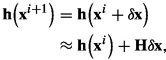(5.12)

where $${\mathbf {H}}$$ follows the definition from Eq. (4.12) and

\begin{aligned} {\mathbf {x}}^{i+1}= {\mathbf {x}}^{i}+ {\delta {\mathbf {x}}}. \end{aligned}
(5.13)

With this, we can write the model residual in Eq. (5.8) as

\begin{aligned} \begin{aligned} {\mathbf {x}}_k^{i+1}-{\mathbf {m}}\bigl ({\mathbf {x}}_{k-1}^{i+1}\bigr )&\approx {\mathbf {x}}_k^{i+1}- {\mathbf {m}}\bigl ({\mathbf {x}}_{k-1}^{i}\bigr ) - {\mathbf {M}}_{k-1} {\delta {\mathbf {x}}}_{k-1} \\&= {\mathbf {x}}_k^{i+1}- {\mathbf {x}}_k^{i}+{\mathbf {x}}_k^{i}- {\mathbf {m}}\bigl ({\mathbf {x}}_{k-1}^{i}\bigr ) - {\mathbf {M}}_{k-1} {\delta {\mathbf {x}}}_{k-1} \\&= {\delta {\mathbf {x}}}_k - {\mathbf {M}}_{k-1} {\delta {\mathbf {x}}}_{k-1} + \boldsymbol{\xi }_k^{i}, \end{aligned} \end{aligned}
(5.14)

where for the time steps $$k=1,\ldots ,K$$

\begin{aligned} \boldsymbol{\xi }_k^{i}= {\mathbf {x}}_k^{i}- {\mathbf {m}}\bigl ({\mathbf {x}}_{k-1}^{i}\bigr ) , \end{aligned}
(5.15)

is the deviation of the model trajectory from the exact model solution. For the increment’s initial-condition term we define as before $$\boldsymbol{\xi }_0^{i}= {\mathbf {x}}_0^{i}- {\mathbf {x}}_0^\mathrm {f}$$. The innovations are

\begin{aligned} \boldsymbol{\eta }^{i}= {\mathbf {d}}- {\mathbf {h}}\bigl ({\mathbf {x}}^{i}\bigr ). \end{aligned}
(5.16)

We insert these definitions into the cost function for the generalized inverse in Eq. (5.8) to get the inner cost function as

The weak-constraint incremental cost function

\begin{aligned} \mathcal {J}({\delta {\mathbf {x}}})&= \frac{1}{2}\bigl ({\delta {\mathbf {x}}}_0 - \boldsymbol{\xi }_0^{i}\bigr )^\mathrm {T}{\mathbf {C}}_{x_0x_0}^{-1} \bigl ({\delta {\mathbf {x}}}_0 - \boldsymbol{\xi }_0^{i}\bigr ) \nonumber \\&+\frac{1}{2}\bigl ({\mathbf {H}}^{i}{\delta {\mathbf {x}}}-\boldsymbol{\eta }^{i}\bigr )^{\mathrm {T}}\,{\mathbf {C}}_\textit{dd}^{-1}\, \bigl ({\mathbf {H}}^{i}{\delta {\mathbf {x}}}-\boldsymbol{\eta }^{i}\bigr ) \\&+ \frac{1}{2}\sum _{r=1}^{K}\sum _{s=1}^{K}\bigl ({\delta {\mathbf {x}}}_r - {\mathbf {M}}_{r-1} {\delta {\mathbf {x}}}_{r-1} +\boldsymbol{\xi }_r^{i}\bigr )^\mathrm {T}{\mathbf {C}}_\textit{qq}^{-1}(r,s) \bigl ({\delta {\mathbf {x}}}_{s} - {\mathbf {M}}_{s-1} {\delta {\mathbf {x}}}_{s-1} +\boldsymbol{\xi }_s^{i}\bigr ) \nonumber . \end{aligned}
(5.17)

Since the model is linear in the inner loop, a Gaussian prior for the initial condition leads to a Gaussian prior on the model states in the whole time window. Hence, we can again assume a Gaussian prior for the unknown state increment $${\delta {\mathbf {x}}}= {\mathbf {x}}^{i+1}- {\mathbf {x}}^{i}$$ for the model’s initial condition and the model solution at all instants in the assimilation window.

## 4 Minimizing the Cost Function for the Increment

Taking the gradient of the cost function in Eq. (5.17) to the model state $${\delta {\mathbf {x}}}_k$$ gives, for $$k \ne 0$$

\begin{aligned} \begin{aligned} \nabla _{{\delta {\mathbf {x}}}_k} \mathcal {J}({\delta {\mathbf {x}}})&= {{\mathbf {H}}_k^{i}}^{\mathrm {T}}{\mathbf {C}}_\textit{dd}^{-1} \bigl ({\mathbf {H}}^{i}{\delta {\mathbf {x}}}-\boldsymbol{\eta }^{i}\bigr ) \\&+ \sum _{s=1}^K{\mathbf {C}}_\textit{qq}^{-1}(k,s)\bigl ({\delta {\mathbf {x}}}_s-{\mathbf {M}}_{s-1}^{i}{\delta {\mathbf {x}}}_{s-1}+\boldsymbol{\xi }_s^{i}\bigr ) \\&- {{\mathbf {M}}_k^{i}}^\mathrm {T}\sum _{s=1}^K{\mathbf {C}}_\textit{qq}^{-1}(k+1,s)\bigl ({\delta {\mathbf {x}}}_s-{\mathbf {M}}_{s-1}^{i}{\delta {\mathbf {x}}}_{s-1}+\boldsymbol{\xi }_s^{i}\bigr ). \end{aligned} \end{aligned}
(5.18)

From this equation, we can define an adjoint vector for each time step as

\begin{aligned} {\delta \boldsymbol{\lambda }}_k = \sum _{s=1}^K {\mathbf {C}}_\textit{qq}^{-1}(k,s) \bigl ({\delta {\mathbf {x}}}_s-{\mathbf {M}}_{s-1}^{i}{\delta {\mathbf {x}}}_{s-1}+\boldsymbol{\xi }_s^{i}\bigr ), \end{aligned}
(5.19)

such that we can write Eq. (5.18) as

\begin{aligned} \begin{aligned} \nabla _{{\delta {\mathbf {x}}}_k} \mathcal {J}({\delta {\mathbf {x}}})&= {{\mathbf {H}}_k^{i}}^{\mathrm {T}}{\mathbf {C}}_\textit{dd}^{-1} \bigl ({\mathbf {H}}^{i}{\delta {\mathbf {x}}}-\boldsymbol{\eta }^{i}\bigr ) + {\delta \boldsymbol{\lambda }}_k - {{\mathbf {M}}_{k}^{i}}^{\mathrm {T}}{\delta \boldsymbol{\lambda }}_{k+1} . \end{aligned} \end{aligned}
(5.20)

For the initial time $$k=0$$ we find for the gradient

\begin{aligned} \begin{aligned} \nabla _{{\delta {\mathbf {x}}}_0} \mathcal {J}({\delta {\mathbf {x}}})&= {\mathbf {C}}_{x_0x_0}^{-1} \bigl ({\delta {\mathbf {x}}}_0 - \boldsymbol{\xi }_0^{i}\bigr ) - {{\mathbf {M}}_0^{i}}^{\mathrm {T}}{\delta \boldsymbol{\lambda }}_{1}\\&= {\mathbf {C}}_{x_0x_0}^{-1} \bigl ({\delta {\mathbf {x}}}_0 - \boldsymbol{\xi }_0^{i}\bigr ) - {\delta \boldsymbol{\lambda }}_{0} , \end{aligned} \end{aligned}
(5.21)

where we used the definition of the adjoint variable (5.19).

If we now set all gradients of the cost function to zero, we find the Euler-Lagrange equations, which comprise a two-point boundary value problem in time consisting of the forward model with the initial condition for the increments $${\delta {\mathbf {x}}}$$

Forward model

\begin{aligned} {\delta {\mathbf {x}}}_0&= \boldsymbol{\xi }_0^{i}+ {\mathbf {C}}_{x_0 x_0} {\delta \boldsymbol{\lambda }}_{0}, \end{aligned}
(5.22)
\begin{aligned} {\delta {\mathbf {x}}}_k - {\mathbf {M}}_{k-1}^{i}{\delta {\mathbf {x}}}_{k-1}&= -\boldsymbol{\xi }_k^{i}+ \sum _{s=1}^K{\mathbf {C}}_\textit{qq}(k,s) {\delta \boldsymbol{\lambda }}_s , \end{aligned}
(5.23)

and the backward model for the adjoint variable $${\delta \boldsymbol{\lambda }}$$

Backward model

\begin{aligned} {\delta \boldsymbol{\lambda }}_{K+1}&= {\mathbf {0}}, \end{aligned}
(5.24)
\begin{aligned} {\delta \boldsymbol{\lambda }}_k - {{\mathbf {M}}_k^{i}}^{\mathrm {T}}{\delta \boldsymbol{\lambda }}_{k+1}&= - {{\mathbf {H}}_k^{i}}^{\mathrm {T}}{\mathbf {C}}_\textit{dd}^{-1} \bigl ({\mathbf {H}}^{i}{\delta {\mathbf {x}}}-\boldsymbol{\eta }^{i}\bigr ). \end{aligned}
(5.25)

The Eqs. (5.22)–(5.25) define the minimizing solution of the variational problem defined by the incremental cost function in Eq. (5.17). Due to the coupling of these equations, an iterative solution procedure is a natural choice. Note that by setting $${\mathbf {C}}_\textit{qq}=0$$, we decouple the forward model integration from the adjoint variable, leading to the SC-4DVar method discussed above. In this case, we restrict ourselves to iteratively solving for the initial conditions as the only unknown. As an alternative to iterative solution methods, the representer method decouples the forward and backward models. We will discuss this approach in the following section.

## 5 Observation Space Formulation

In the following, we explore that the observation space is typically much smaller than the state space, which is even more true for the weak-constraint case due to the larger state vector. While the problem size grows dramatically in the state-space from the strong-constraint formulation to the weak-constraint 4DVar, it does not grow in the observation space. Hence, solving the weak-constraint problem in observation space is likely more efficient, as was realized and discussed by Bennett (1992). He formulated a solution method for the weak-constraint problem called the representer method. While the original representer method is illustrative, it is not efficient with many observations, as the method requires a backward (adjoint) and forward model integration for each measurement. A later variant by Egbert et al. (1994) avoids this problem. We will discuss the representer method below as it is highly efficient for linear inverse problems and provides for further insight into the data assimilation problem.

### 5.1 Original Representer Method

The representer method by Bennett (1992) exploits the “weak coupling” in the Euler-Lagrange Eqs. (5.22)–(5.25) through the measurement term in Eq. (5.24). Let’s assume a solution of the form

\begin{aligned} {\delta {\mathbf {x}}}&= {\delta {\mathbf {x}}}^{\mathrm {f}} + \sum _{p=1}^m b_p {\mathbf {r}}_p = {\delta {\mathbf {x}}}^{\mathrm {f}} + {\mathbf {R}}{\mathbf {b}}, \end{aligned}
(5.26)
\begin{aligned} {\delta \boldsymbol{\lambda }}&= {\delta \boldsymbol{\lambda }}^{\mathrm {f}} + \sum _{p=1}^m b_p {\mathbf {s}}_p = {\delta \boldsymbol{\lambda }}^{\mathrm {f}} + {\mathbf {S}}{\mathbf {b}}. \end{aligned}
(5.27)

Here we assume $${\delta {\mathbf {x}}}^{\mathrm {f}}\ne 0$$ and $${\delta \boldsymbol{\lambda }}^{\mathrm {f}}=0$$ to be a first-guess solution which would result from the case with no “observations”, i.e., no forcing term in Eq. (5.24). In Eqs. (5.26) and (5.27) we assume the solution to equal the first guess plus a linear combination of m representers or influence functions $${\mathbf {r}}_p$$ and their adjoints $${\mathbf {s}}_p$$. There is one representer function for each of the m measurements, and we store them in the m columns of the matrix $${\mathbf {R}}$$. Bennett (1992) showed that this linear combination of representer functions exactly represents the minimizing solution.

Inserting the expressions for $${\delta {\mathbf {x}}}$$ and $${\delta \boldsymbol{\lambda }}$$ in the Euler-Lagrange Eqs. (5.22)–(5.25) gives for the first-guess solution

\begin{aligned} {\delta {\mathbf {x}}}_0^{\mathrm {f}}&= \boldsymbol{\xi }_0^{i}, \end{aligned}
(5.28)
\begin{aligned} {\delta {\mathbf {x}}}_k^{\mathrm {f}} - {\mathbf {M}}_{k-1}^{i}{\delta {\mathbf {x}}}_{k-1}^{\mathrm {f}}&= -\boldsymbol{\xi }_k^{i}, \end{aligned}
(5.29)
\begin{aligned} {\delta \boldsymbol{\lambda }}_{K+1}^{\mathrm {f}}&= 0 , \end{aligned}
(5.30)
\begin{aligned} {\delta \boldsymbol{\lambda }}_k^{\mathrm {f}} - {{\mathbf {M}}_k^{i}}^{\mathrm {T}}{\delta \boldsymbol{\lambda }}_{k+1}^{\mathrm {f}}&= 0. \end{aligned}
(5.31)

For the representers and their adjoints we obtain

\begin{aligned} {\mathbf {R}}_0{\mathbf {b}}&= {\mathbf {C}}_{x_0 x_0} {{\mathbf {M}}_{0}^{i}}^{\mathrm {T}}{\mathbf {S}}_{1}{\mathbf {b}}, \end{aligned}
(5.32)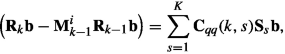(5.33)
\begin{aligned} {\mathbf {S}}_{K+1}{\mathbf {b}}&= {\mathbf {0}}, \end{aligned}
(5.34)
\begin{aligned} {\mathbf {S}}_k{\mathbf {b}}- {{\mathbf {M}}_k^{i}}^{\mathrm {T}}{\mathbf {S}}_{k+1}{\mathbf {b}}&= {{\mathbf {H}}_k^{i}}^{\mathrm {T}}{\mathbf {C}}_\textit{dd}^{-1} \bigl (\boldsymbol{\eta }^{i}- {\mathbf {H}}^{i}( {\delta {\mathbf {x}}}^{\mathrm {f}} + {\mathbf {R}}{\mathbf {b}}) \bigr ). \end{aligned}
(5.35)

The decomposition in Eqs.(5.26) and (5.27) enforce that $${\mathbf {R}}$$ and $${\mathbf {S}}$$ are not functions of $${\mathbf {b}}$$. This can be achieved by defining $${\mathbf {b}}$$ as

\begin{aligned} {\mathbf {b}}= {\mathbf {C}}_\textit{dd}^{-1} \bigl (\boldsymbol{\eta }^{i}- {\mathbf {H}}^{i}( {\delta {\mathbf {x}}}^{\mathrm {f}} + {\mathbf {R}}{\mathbf {b}}) \bigr ), \end{aligned}
(5.36)

such that Eq. (5.35) simplifies to

\begin{aligned} {\mathbf {S}}_k{\mathbf {b}}- {{\mathbf {M}}_k^{i}}^{\mathrm {T}}{\mathbf {S}}_{k+1}{\mathbf {b}}= {{\mathbf {H}}_k^{i}}^{\mathrm {T}}{\mathbf {b}}. \end{aligned}
(5.37)

Since $${\mathbf {b}}\ne 0$$ and acts as a common multiplier in all the Eqs. (5.32, 5.33, 5.34, and 5.37) we can write the following uncoupled system of equations for the representers and their adjoints,

\begin{aligned} {\mathbf {R}}_0&= {\mathbf {C}}_{x_0 x_0} {{\mathbf {M}}_{0}^{i}}^{\mathrm {T}}{\mathbf {S}}_{1}, \end{aligned}
(5.38)
\begin{aligned} {\mathbf {R}}_k - {\mathbf {M}}_{k-1}^{i}{\mathbf {R}}_{k-1}&= \sum _{s=1}^K{\mathbf {C}}_\textit{qq}(k,s) {\mathbf {S}}_s , \end{aligned}
(5.39)
\begin{aligned} {\mathbf {S}}_{K+1}&= {\mathbf {0}}, \end{aligned}
(5.40)
\begin{aligned} {\mathbf {S}}_k - {{\mathbf {M}}_k^{i}}^{\mathrm {T}}{\mathbf {S}}_{k+1}&= {{\mathbf {H}}_k^{i}}^{\mathrm {T}}. \end{aligned}
(5.41)

Here $${{\mathbf {H}}_k^{i}}$$ is the columns of $${\mathbf {H}}^{i}$$ in Eq. (4.12) corresponding to the time k. The matrix $${\mathbf {R}}$$’s columns contain the influence functions $${\mathbf {r}}_s$$, and the matrix $${\mathbf {S}}$$ contains their adjoints.

Thus, a backward-in-time integration of Eqs. (5.40) and (5.41) determines $${\mathbf {S}}$$ and we can then solve for $${\mathbf {R}}$$ by a forward integration of Eqs. (5.38) and (5.39). What remains is then to determine $${\mathbf {b}}$$ from Eq. (5.36), i.e.,

Linear system for the representer coefficients $${\mathbf {b}}$$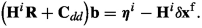(5.42)

Bennett (2002) gives a detailed explanation of how to solve for the representer solution efficiently. First, note that as soon as we have computed $${\mathbf {b}}$$ by solving Eq. (5.42), we can use the definition of $${\mathbf {b}}$$ from Eq. (5.36) and write the adjoint Eq. (5.25) as

Adjoint equation forced by $${\mathbf {b}}$$

\begin{aligned} {\delta \boldsymbol{\lambda }}_k - {{\mathbf {M}}_k^{i}}^{\mathrm {T}}{\delta \boldsymbol{\lambda }}_{k+1} = {{\mathbf {H}}_k^{i}}^{\mathrm {T}}{\mathbf {b}}. \end{aligned}
(5.43)

Thus, knowing $${\mathbf {b}}$$ allows us to compute the solution by one backward integration of Eq. ( 5.43 ) subject to the final condition in Eq. ( 5.24 ), followed by one forward integration of the model with initial condition in Eqs. ( 5.22 ) and ( 5.23 ).

Notice that we do not need to store all the representers and their adjoints. We must only construct the “representer matrix”

\begin{aligned} \boldsymbol{\mathcal {R}}= {\mathbf {H}}{\mathbf {R}} \end{aligned}
(5.44)

that enables us to solve the system in Eq. (5.42).

The representer method has a beautiful property. It shows via its basic construction in Eq. (5.26) that the solution to the linear data-assimilation problem is the first-guess solution plus a linear combination of the representers. Furthermore, we can interpret any representer $${\mathbf {r}}_p$$ as the influence function for measurement p. We construct it as column p of the matrix Eqs. (5.38)–(5.41). Specifically, each measurement generates a forcing field for the adjoint representer at the observation time. This forcing field is propagated back to the initial time via the adjoint representer equations. After that, the adjoint representers $${\mathbf {s}}_p$$ force the forward integration when computing the representers $${\mathbf {r}}_p$$. In this way, we spread out the influence of measurement p over the whole space-time domain. And, as mentioned, the complete solution to the linear problem is the first guess plus a linear combination of these space-time influence functions, one for each measurement.

The solution method outlined above is inefficient as it needs a full adjoint and forward model integration for each observation. On the other hand, the solution method illustrates well the nature of the weak-constraint estimation problem. Note also that the representer solution is the unique minimizing solution of the Euler-Lagrange equations, as first noticed by Bennett (1992). The following section discusses a much more efficient implementation of the representer method. For an application that illustrates some of the representer method’s properties, we refer to the example in Chap. 17.

### 5.2 Efficient Weak-Constraint Solution in Observation Space

In the previous section, we saw that we need to solve for the vector $${\mathbf {b}}$$ from Eq. (5.42), which we write as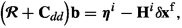(5.45)

using the definition of the representer matrix in Eq. (5.44).

Recall that, as soon as $${\mathbf {b}}$$ is known, we can find the final solution via one backward integration of Eq. (5.43) subject to the condition in Eq. (5.24), followed by an integration of the model in Eqs. (5.22) and (5.23).

Apparently, the definition in Eq. (5.44) requires us to form the representer matrix $$\boldsymbol{\mathcal {R}}$$ to solve the linear system in Eq. (5.45), for which we need to compute all the representers. However, suppose we use an iterative method for solving Eq. (5.45). In that case we only need to calculate products $$(\boldsymbol{\mathcal {R}}+{\mathbf {C}}_\textit{dd}) {\mathbf {v}}$$ for arbitrary vectors $${\mathbf {v}}$$. As $${\mathbf {C}}_\textit{dd}$$ is known, the problem is reduced to computing the product $$\boldsymbol{\mathcal {R}}{\mathbf {v}}= {\mathbf {H}}{\mathbf {R}}{\mathbf {v}}$$.

But, if we write the Eqs. (5.32), (5.33), (5.34), and (5.37) as

\begin{aligned} ({\mathbf {R}}_0{\mathbf {v}})&= {\mathbf {C}}_{x_0 x_0} {{\mathbf {M}}_{0}^{i}}^{\mathrm {T}}({\mathbf {S}}_{1}{\mathbf {v}}), \end{aligned}
(5.46)
\begin{aligned} ({\mathbf {R}}_k{\mathbf {v}})&= {\mathbf {M}}_{k-1}^{i}({\mathbf {R}}_{k-1}{\mathbf {v}}) + \sum _{s=1}^K{\mathbf {C}}_\textit{qq}(k,s) ({\mathbf {S}}_s{\mathbf {v}}) , \end{aligned}
(5.47)
\begin{aligned} ({\mathbf {S}}_{K+1}{\mathbf {v}})&= {\mathbf {0}}, \end{aligned}
(5.48)
\begin{aligned} ({\mathbf {S}}_k{\mathbf {v}})&= {{\mathbf {M}}_k^{i}}^{\mathrm {T}}({\mathbf {S}}_{k+1}{\mathbf {v}}) + {{\mathbf {H}}_k^{i}}^{\mathrm {T}}{\mathbf {v}}, \end{aligned}
(5.49)

we can compute the product $${\mathbf {c}}={\mathbf {R}}{\mathbf {v}}$$ by one backward integration of Eq. (5.49) subject to the final condition (5.48) to obtain the field $$\boldsymbol{\psi }={\mathbf {S}}{\mathbf {v}}$$, followed by a forward integration of the Eq. (5.47) from the initial condition in Eq. (5.46). Thus, we rewrite these equations as

\begin{aligned} {\mathbf {c}}_0&= {\mathbf {C}}_{x_0 x_0} {{\mathbf {M}}_{0}^{i}}^{\mathrm {T}}\boldsymbol{\psi }_{1}, \end{aligned}
(5.50)
\begin{aligned} {\mathbf {c}}_k&= {\mathbf {M}}_{k-1}^{i}{\mathbf {c}}_{k-1} + \sum _{s=1}^K{\mathbf {C}}_\textit{qq}(k,s) \boldsymbol{\psi }_s , \end{aligned}
(5.51)
\begin{aligned} \boldsymbol{\psi }_{K+1}&= {\mathbf {0}}, \end{aligned}
(5.52)
\begin{aligned} \boldsymbol{\psi }_k&= {{\mathbf {M}}_k^{i}}^{\mathrm {T}}\boldsymbol{\psi }_{k+1} + {{\mathbf {H}}_k^{i}}^{\mathrm {T}}{\mathbf {v}}. \end{aligned}
(5.53)

By measuring this solution, we find for any nonzero vector $${\mathbf {v}}$$

\begin{aligned} {\mathbf {H}}{\mathbf {c}}= {\mathbf {H}}{\mathbf {R}}{\mathbf {v}}= \boldsymbol{\mathcal {R}}{\mathbf {v}}, \end{aligned}
(5.54)

which is precisely the matrix-vector product we need to compute.

This algorithm by Egbert et al.  (1994) calculates the product $$\boldsymbol{\mathcal {R}}{\mathbf {v}}$$ without knowing $$\boldsymbol{\mathcal {R}}$$ by performing one backward and one forward model integration. Thus, it is possible to solve Eq. (5.45) iteratively for $${\mathbf {b}}$$, using only two model integrations per iteration. Isn’t that an astonishing result? In the following two sections, we introduce an iterative solver to illustrate the methodology. We also discuss an efficient approach for computing the convolutions of the model- and initial-error covariances with the adjoint variable.

We present an example algorithm for solving the weak-constraint inverse problem in Algorithm 4. Here we combine the outer incremental Gauss–Newton iterations with the representer method for solving the linear inverse problems for the increments. Note that it is also possible to use the Algorithm 4 to solve the strong constraint variational problem by setting $${\mathbf {C}}_\textit{qq}\equiv 0$$.

#### 5.2.1 Iterative Equation Solver

We can illustrate the iterative procedure for solving Eq. (5.45) by using a steepest descent method. Let’s write the linear system in Eq. (5.45) as

\begin{aligned} {\mathbf {C}}{\mathbf {b}}= \boldsymbol{\eta }. \end{aligned}
(5.55)

Solving Eq. (5.55) is equivalent to minimizing the functional

\begin{aligned} \phi ({\mathbf {b}}) = \frac{1}{2}{\mathbf {b}}^\mathrm {T}{\mathbf {C}}{\mathbf {b}}- {\mathbf {b}}^\mathrm {T}\boldsymbol{\eta }, \end{aligned}
(5.56)

\begin{aligned} \nabla _{\mathbf {b}}\phi ({\mathbf {b}}) = \boldsymbol{\rho }= {\mathbf {C}}{\mathbf {b}}- \boldsymbol{\eta }. \end{aligned}
(5.57)

We can then minimize the cost function in Eq. (5.56) iteratively by using an iterative approach, .e.g.,

\begin{aligned} {\mathbf {b}}^{i+1}= {\mathbf {b}}^{i}- \gamma \boldsymbol{\rho }, \end{aligned}
(5.58)

where $$\gamma = \boldsymbol{\rho }^\mathrm {T}\boldsymbol{\rho }/ \boldsymbol{\rho }^\mathrm {T}{\mathbf {C}}\boldsymbol{\rho }$$ is the optimal steepest-descent steplength. Of course, in real problems we should introduce preconditioning or use a conjugate gradient method to speed up the convergence.

#### 5.2.2 Fast Computation of the Error Terms

The final issue of using the representer method for real-sized problems, is how to compute the discrete convolutions in the model error term in Eqs. (5.23) and (5.51), and in Algorithm 4. The direct computation of matrix-vector multiplications in these terms becomes too computationally demanding for real problems. Even for the initial conditions, the multiplication $${\mathbf {C}}_{x_0x_0} {\delta \boldsymbol{\lambda }}_0$$ requires $$n^2$$ operations, with n being the size of the model state vector.

The approach taken by Bennett (1992) was to use a Gaussian covariance where the Fourier transform is known and diagonal and use fast Fourier transforms to compute the multiplication efficiently in Fourier space at a cost proportional to $$n\ln n$$. Bennett (2002) pointed out that with certain assumptions on the “shape” of the covariance matrix $${\mathbf {C}}_\textit{qq}(k,s)$$, even more efficient methods exist.

Let’s assume the covariance to be isotropic and separable in space and time with Gaussian covariance in space and exponential covariance in time, i.e.,(5.59)

where $$x_i$$ and $$x_j$$ are two spatial model gridpoints, and $$t_r$$ and $$t_s$$ are two time steps. We also define the decorrelation lengths $$r_x$$ in space and $$\tau$$ in time. Then, for a model with spatial dimension d we must compute the following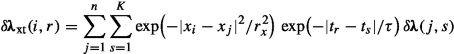(5.60)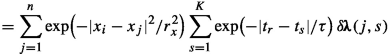(5.61)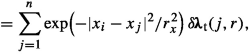(5.62)

where we defined

\begin{aligned} {\delta \boldsymbol{\lambda }}_\text {t}(j,r) = \sum _{s=1}^K \exp \bigl (-|t_r-t_s|/\tau \bigr )\, {\delta \boldsymbol{\lambda }}(j,s) . \end{aligned}
(5.63)

The solution procedure proposed by Bennett (2002) uses that the expression in Eq. (5.63) when written in the continuous form in time

\begin{aligned} {\delta \lambda }_\text {t}(x_j,t) = \int _0^T \exp \bigl (-|t-t'|/\tau \bigr ) \,{\delta \lambda }\bigl (x_j,t'\bigr ) \,\textit{dt}' , \end{aligned}
(5.64)

for each value of $$x_j$$, is the solution of a two-point boundary value problem in time (Bennett 2002, see pages 65–66),

\begin{aligned} \frac{{\partial }^2 {\delta \lambda }_\text {t}}{\partial {t}^2} - \frac{1}{\tau ^2} {\delta \lambda }_\text {t}&= -\frac{2{\delta \lambda }(x_j,\tau )}{\tau } \end{aligned}
(5.65)
\begin{aligned} \frac{\partial {\delta \lambda }_\text {t}}{\partial t} - \frac{1}{\tau } {\delta \lambda }_\text {t}&=0 \quad \text{ for } t=0 \end{aligned}
(5.66)
\begin{aligned} \frac{\partial {\delta \lambda }_\text {t}}{\partial t} + \frac{1}{\tau } {\delta \lambda }_\text {t}&=0 \quad \text{ for } t=T. \end{aligned}
(5.67)

We can solve this one-dimensional boundary value problem for each of the $$j=1,n$$ functions $${\delta \boldsymbol{\lambda }}(x_j,\tau )$$ to obtain $${\delta \boldsymbol{\lambda }}_\text {t}(j,r)$$.

Furthermore, as soon as we have $${\delta \lambda }_\text {t}(x,t)$$, or in discrete form $${\delta \boldsymbol{\lambda }}_\text {t}(j,r)$$, then the expression in Eq. (5.62), becomes in continous form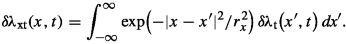(5.68)

We will now use that the variable $$\theta (x,s)$$ defined as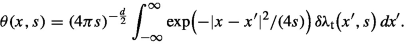(5.69)

is the solution of the heat equation

\begin{aligned} \frac{\partial \theta }{\partial s} = \nabla ^2 \theta , \end{aligned}
(5.70)

subject to the initial condition

\begin{aligned} \theta (x,s=0) = {\delta \lambda }_\text {t}(x,t), \end{aligned}
(5.71)

(Bennett, 2002; Wikipedia, 2022). Thus , $${\delta \lambda }_\text {xt}(x,t)$$ becomes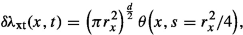(5.72)

so, we just need to integrate Eq. (5.70) from $$s=0$$ to $$s=r_x^2/4$$ to get $$\theta (s)$$ and hence $${\delta \lambda }_\text {xt}(x,t)$$ from Eq. (5.72).

For the initial conditions, we can compute the convolution in Eq. (5.68) at a cost proportional to n, or precisely n times the required number of pseudo time-steps needed when solving the diffusion Eq. (5.70) from $$s=0$$ to $$s=r_x^2/4$$. We need to solve the diffusion Eq. (5.70) for each time step for the model error term. Thus the computational cost becomes proportional to nK. Additionally, we need to solve the boundary value problem in Eqs. (5.65)–(5.67) nK times, one time for each model gridpoint in space and time.

Bennett (2002) provides a detailed description of this algorithm, and we note that Courtier (1997) also discusses the incremental weak constraint method in conjunction with WC-4DVar. The US Navy uses an operational implementation of the representer method with an ocean model  (Souopgui et al., 2017). They also have a dormant representer implementation for their atmospheric model.